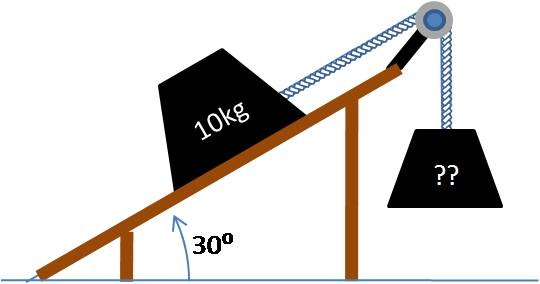# Thread: Force normal and force applied.

1. ## Force normal and force applied.

A 10 kg block is at rest on a frictionless incline at $30^\circ$ to the horizontal, held in place by a rope attached to a mass hanging off the end of the incline.

What is the mass of the block at the other end of the rope?I think mass of the block at the other end of rope is 10kg. Is my answer correct? What do you think?

2. ## Re: Force normal and force applied.Originally Posted by VinodA 10 kg block is at rest on a frictionless incline at $30^\circ$ to the horizontal, held in place by a rope attached to a mass hanging off the end of the incline.

What is the mass of the block at the other end of the rope?I think mass of the block at the other end of rope is 10kg. Is my answer correct? What do you think?
Why do you say that?

The force holding the unknown mass up is going to be the same as the force (due to gravity) pulling the 10 kg block down the incline. What is the magnitude of that force?

-Dan

3. ## Re: Force normal and force applied.Originally Posted by topsquarkWhy do you say that?

The force holding the unknown mass up is going to be the same as the force (due to gravity) pulling the 10 kg block down the incline. What is the magnitude of that force?

-Dan

49N is the $F_{applied}$. So what would be a mass of unknown block?

4. ## Re: Force normal and force applied.

5kg mass of block